Passive sensors

A sensor is a device that detects or measures a physical quantity and converts it into another physical quantity (like movement converted to resistance for example). In this section an overview of the basic passive sensors. A passive sensor is a sensor that does not need power in order to work.

Switch (status on, off)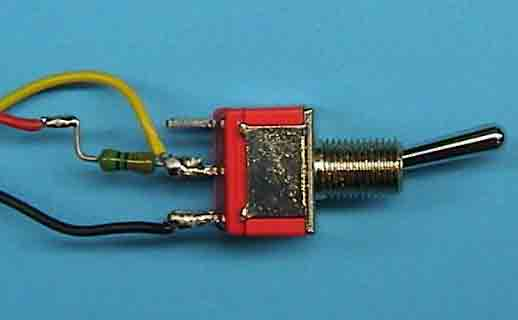The resistive passive sensor changes value (electrical) when the physical quantity (e.a. temperature, force, light etc) changes. These sensors can (generally) be used in combination with an extra resistor, like described above.
Switches  The most common sensor is a switch. A switch is actually a resistor with two states: infinite and zero. When a switch has to be connected to the analog input of a sensor-interface, the same series resistor as mentioned before is needed.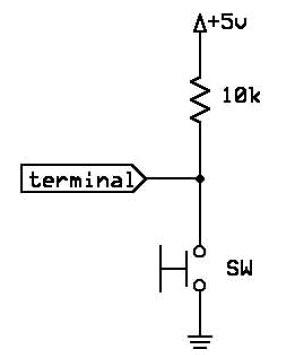A good value of the series resistor R1 will be between 10K-50K. In rest situation (switch is not pushed), the output will be +5V. When the switch is pushed, the resistance will be zero (shortcut to gnd) and the output voltage will be 0V.

Potentiometers (rotation, displacement)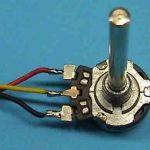A potentiometer is a well known sensor, which can be found in almost every electronic device. A potentiometer is a resistor that changes value when the position is changed; i.e. the volume button of your radio, the fader of an audio-mixer etc. The potentiometer is the only resistive sensor, which doesn’t necessarily needs a series resistor. The potentiometer itself can be seen as two different resistors.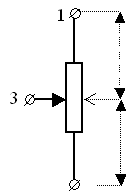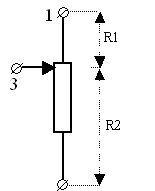It has a resistance element that is attached by three contacts, or terminals. The two ends of the resistor are directly connected to two terminals. The third one (the middle) is usually a moveable terminal that slides across the resistance element, effectively dividing it into two resistors; for this reason it is called a voltage divider.

A few other examples of potentiometers (displacement measurement):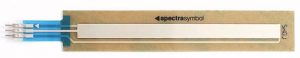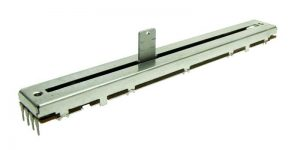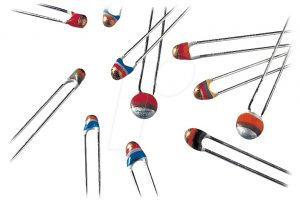NTC, PTC sensors (temperature)
There is a wide range of temperature sensors. In general these type of sensors change resistor value, when the temperature changes. The simplest form of temperature sensors are NTC or PTC. This stands for Negative Temperature Coefficient or Positive Temperature Coefficient. These components have two connections (like a resistor). In case of a NTC, the value of the resistor will decrease if the temperature rises. The PTC will do the opposite.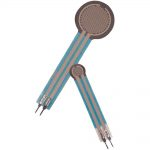Force Sensing resistors  (pressure, displacement)
Nicknamed “the electronic gas pedal”, an FSR is a tactile sensor which responds with variable resistance. The harder you press on this thick film device, the more its resistance drops.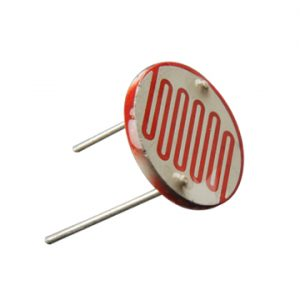Light sensors (light)
Light sensors are resistors that change resistance when the intensity of the (visible) light changes. The most common form is the LDR. This stands for Light Dependent Resistor.

Piezo element (pressure)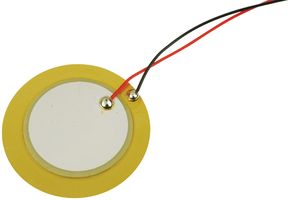A piezo sensor/transducer is a passive element and works both ways. When a pressure is applied (sound) an electric voltage is generated. If you apply an ac avoltage to the piezo element, a loud beep will be generated (transducer).

DC motor (rotation speed)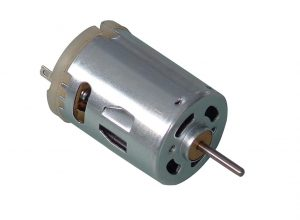A small DC-engine can be used as a sensor, sensing the speed of the rotation for example. It works as a dynamo, generating voltage (energy) when rotated.